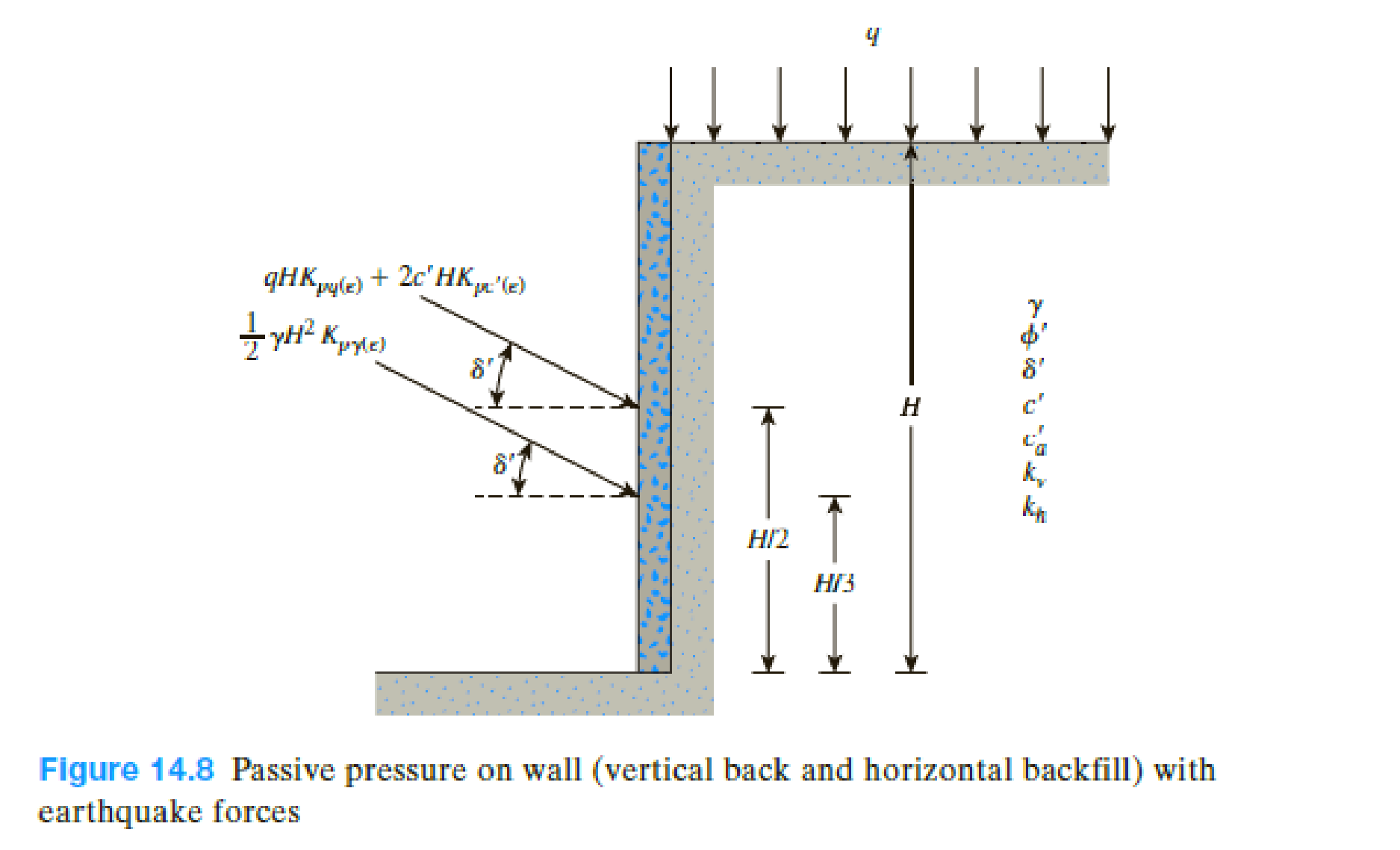Chapter 14, Problem 14.11PPrinciples of Geotechnical Enginee...

9th Edition
Braja M. Das + 1 other
ISBN: 9781305970939

Solutions

Chapter
SectionPrinciples of Geotechnical Enginee...

9th Edition
Braja M. Das + 1 other
ISBN: 9781305970939
Textbook Problem

A retaining wall located in an earthquake zone has a vertical back face and a horizontal backfill (Figure 14.8). The wall is also subjected to a surcharge loading of magnitude, q = 95 kN/m2. Determine the magnitude and location of the passive force, Ppe, due to earthquake conditions. Given: γ = 18 kN/m3, ϕ' = 40°, δ' = 20°, c' = 30 kN/m2, c ′ a = 0 , H = 7 m, and kv = kh = 0.2.To determine

Find the magnitude and location of the passive force Ppe due to earthquake conditions.

Explanation

Given information:

The magnitude of surcharge loading (q) is 95kN/m2.

The unit weight γ of the backfill is 18kN/m3.

The height (H) of the retaining wall is 7.0 m.

The soil friction angle ϕ is 40°.

The angle of wall friction δ is 20°.

The cohesion c of the soil is 30kN/m2.

The soil-wall interfall adhesion ca is 0.

The horizontal inertial kh force is 0.2.

The vertical inertial kv force is 0.2.

Calculation:

Determine the ratio of angle of wall friction to the soil friction angle.

δϕ=20°40°=0.5

Determine the ratio of soil-wall interfall adhesion to the cohesion.

cac=030=0

Determine the magnitude of the passive force due to earthquake conditions using the formula.

Ppe=[12γH2Kpγ(e)+qHKpq(e)+2cHKpc(e)]1cosδ (1)

Here, Kpγ(e), Kpq(e), and Kpc(e) are the passive earth-pressure coefficients in the normal direction.

Refer Figure (14.9b) “Variation of Kpγ(e) (a) δϕ=1; (b)” in the text book.

For δϕ=0.5;

Take the value of Kpγ(e) as 6.75.

Refer Figure (14.10b) “Variation of Kpγ(e) (a) δϕ=1; (b)” in the text book.

For δϕ=0.5;

Take the value of Kpq(e) as 6.67.

Refer Table (14.7) “Variation of Kpc(e)” in the text book.

For the ratio of angle of wall friction to the soil friction angle is 0.5.

The value of Kpc(e) is 4.33

Substitute 18kN/m3 for γ, 7.0 m for H, 6.75 for Kpγ(e), 95kN/m2 for q, 6.67 for Kpq(e), 30kN/m2 for c, 4.33 for Kpc(e), and 20° for δ in Equation (1).

Ppe=[12(18)(72)(6.75)+95(7)(6.67)+2(30)(7)(4.33)]1cos20°=9,823kN/m

Thus, the magnitude of the passive force Ppe due to earthquake conditions is 9,823kN/m_.

Determine the unit weight of the passive force Ppe using the formula.

UnitweightofPpe=1cosδ[12γH2Kpγ(e)]

Substitute 20° for δ, 18kN/m3 for γ, 7

Still sussing out bartleby?

Check out a sample textbook solution.

See a sample solution

The Solution to Your Study Problems

Bartleby provides explanations to thousands of textbook problems written by our experts, many with advanced degrees!

Get Started

Name the engine operating conditions that most affect ignition timing requirements.

Automotive Technology: A Systems Approach (MindTap Course List)

List and describe the different types of databases.

Database Systems: Design, Implementation, & Management

What are the main components of a database system?

Database Systems: Design, Implementation, & Management

What are cryptography and cryptanalysis?

Principles of Information Security (MindTap Course List)

What are the two major benefits of titanium?

Precision Machining Technology (MindTap Course List)

Knowing that the resultant of the two forces is directed along AB, determine F2.

International Edition---engineering Mechanics: Statics, 4th Edition

What is a task? What is an event? What is a milestone?

Systems Analysis and Design (Shelly Cashman Series) (MindTap Course List)

What steps can you take to detect keyboard monitoring software?

Enhanced Discovering Computers 2017 (Shelly Cashman Series) (MindTap Course List)